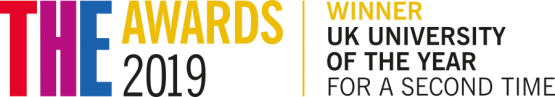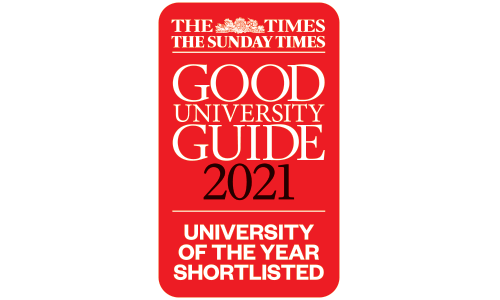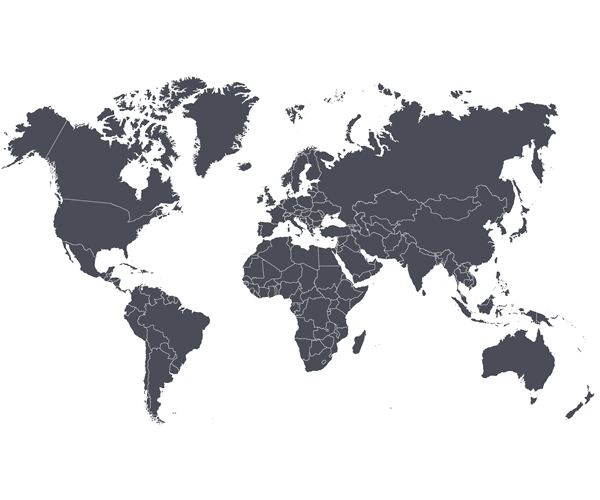# BSc HonsMathematics with Teaching (International)

## Key facts

• Open to international applicants only

• Ranked: top 10 in the UK for Education and Mathematics (The Complete University Guide 2022)

## Study with us

• combine mathematics with teaching methods and practice to prepare you to be a mathematics teacher in secondary schools
• curriculum specifically designed for international students
• learn with Scotland’s largest provider of Initial Teacher Education

## Why this course?

This joint Honours degree covers the curriculum of the BSc Honours Mathematics degree and you’ll also study pedagogy and aspects of education, that will prepare you to become a mathematics teacher in secondary schools.## What you'll study

### Years 1 & 2

You’ll follow the same curriculum as the BSc (Honours) in Mathematics and will study core mathematical methods and classes in calculus, geometry, applied analysis, mechanics, analysis, and probability and statistics. In addition to these compulsory classes, you will also have the opportunity to choose elective classes.

### Year 3

You’ll choose to study from a range of Mathematics & Statistics classes from one or more of the specialist application areas.

### Year 4

In this year you will take classes that develop the pedagogy of teaching.## Course content

##### Introduction to Calculus

You'll study the basic concepts and standard methods of mathematical notation and proof, polynomial equations and inequalities, sequences and series, functions, limits and continuity, differentiation and integration.

##### Applications of Calculus

The fundamental concepts of calculus (differentiation and integration) presented in Applications of Calculus will be examined in more detail, extended to a larger class of functions by means of more sophisticated methods, including an introduction to complex numbers and variables, all demonstrated in application to practical problems including solving basic first and second-order differential equations.

##### Geometry & Algebra with Applications

This class will introduce you to vectors and matrices, along with the idea of mathematical modelling through their application to real-world problems.

##### Statistics & Data Presentation

Some basic ideas and techniques of statistics will be presented while introducing some essential study skills, allowing you to develop and practice personal and technical skills e.g. self-study, teamwork, analysing data, writing reports and making presentations.

##### Applying Mathematics 1

You’ll be introduced to elementary number theory with interesting modern applications.  Codes and encryption:  problem description and motivation.  Mathematical background:  natural numbers and integers, factorisation, proof by induction, highest common factor, lowest common multiple, Euclidean Algorithm, prime numbers and the Fundamental Theorem of Arithmetic, Diophantine equations, modular arithmetic, congruence mod n, solving equations in Zn, Euler's phi-function and theorem.  Applications to International Standard Book Numbers, Universal Product Codes, affine and exponential ciphers, and the RSA cryptosystem.

##### Applying Mathematics 2

To introduce you to areas of mathematics (graph theory) not usually met in school or college courses.  Graph theory: Problem description and motivation (e.g. Konigsberg Bridge, three utilities problem, the travelling salesman problem).  Graphs, multigraphs, isomorphic graphs, sub-graphs, paths, trails, circuits and cycles, Eulerian and Hamiltonian graphs, weighted graphs, minimal spanning trees, critical path analysis, planar graphs, Euler’s formula, Colouring of Graphs, Digraphs, Network Flows, and Social Network Analysis. Brief introduction to network science.

##### Linear Algebra & Differential Equations

This class will introduce you to the basic ideas of linear algebra, such as matrices and determinants, vector spaces, bases, eigenvalues and eigenvectors. You'll study various standard methods for solving ordinary differential equations and understand their relevance.

Basic ideas, techniques and results for calculus of two and three variables, along with differentiation and integration over curves, surfaces and volumes of both scalar and vector fields will be presented.

##### Applicable Analysis

This class will give a rigorous treatment of convergence of sequences and infinite series of real numbers and of continuity, differentiability and integrability of functions of a real variable, and will illustrate the importance of these concepts in the analysis of problems arising in applications.

##### Probability & Statistical Inference

Presentation of the basic concepts of probability theory and statistical inference will be covered to provide you with the tools to appropriately analyse a given data set and effectively communicate the results of such analysis.

##### Introduction to Newtonian Mechanics

This class will develop your appreciation of the basic concepts of force, momentum and energy, and of Newton’s Laws of Motion and will equip you to apply these concepts to model physical systems, in particular the orbital motion of bodies.

##### Mathematical & Statistical Computing

This class will introduce you to the R computing environment. It'll enable you to use R to import data and perform statistical tests, allow you to understand the concept of an algorithm and what makes a good algorithm and will equip you for implementing simple algorithms in R.

### Compulsory classes

##### Differential Equations

We'll introduce you to analytical methods for solving ordinary and partial differential equations so you'll develop an understanding along with technical skills in this area.

##### Inference & Regression Modelling

This class will:

• review the concepts of probability distributions and how to work with these;
• present approaches to parameter estimation, focusing on maximum likelihood estimation, bootstrap estimation, and properties of estimators;
• present hypothesis testing procedures, including classical likelihood ratio tests and computer-based methods for testing parameter values, and goodness-of-fit tests;
• introduce and provide understanding of the least squares multiple regression model, general linear model, transformations and variable selection procedures; and
• present use of R functions for regression and interpretation of R output

### Optional classes

##### Linear Algebra

You’ll be introduced to basic algebraic structures, with particular emphasis on those pertaining to finite dimensional linear spaces and deepen your understanding of linear mappings. You’ll also be introduced to inner product spaces and bilinear forms.

##### Mechanics of Rigid Bodies & Fluids

This class will:

• convey the generalisation of the mechanics of single-particle systems to many-particle systems;
• convey the central ideas of a continuum description of material behaviour and to understand relevant constraints;
• ground students in the basic principles governing three-dimensional motions of rigid bodies;
• and convey how the ideas of continuum theory are applied to static and inviscid fluids
##### Modelling & Simulation with Applications to Financial Derivatives

You’ll be provided with an introduction to ideas in mathematics and statistics that can be used to model real systems, with an emphasis on the valuation of financial derivatives. This module places equal emphasis on deterministic analysis (calculus, differential equations) and stochastic analysis (Brownian motion, birth and death processes). In both cases, in addition to theoretical analysis, appropriate computational algorithms are introduced. The first half of the class introduces general modelling and simulation tools, and the second half focuses on the specific application of valuing financial derivatives, including the celebrated Black-Scholes theory.

##### Applicable Analysis 3

This class will present the main results in Functional Analysis, give you an introduction to linear operators on Banach and Hilbert spaces and study applications to integral and differential equations.

##### Statistical Modelling & Analysis

This class will provide you with a range of applied statistical techniques that can be used in professional life.

##### Fluids & Waves

You'll be introduced to the theory of Newtonian fluids and its application to flow problems and the dynamics of waves on water and in other contexts.

##### Finite Element Methods for Boundary Value Problems & Approximation

You'll be presented with the basic theory and practice of finite element methods and polynomial and piecewise polynomial approximation theory.

##### Applied Statistics in Society

You'll be introduced to a range of modern statistical methods and practices used in industry, commerce and research, and will develop skills in your application and presentation.

##### Mathematical Biology & Marine Population Modelling

You’ll learn the application of mathematical models to a variety of problems in biology, medicine, and ecology. It'll show the application of ordinary differential equations to simple biological and medical problems, the use of mathematical modelling in biochemical reactions, the application of partial differential equations in describing spatial processes such as cancer growth and pattern formation in embryonic development, and the use of delay-differential equations in physiological processes. The marine population modelling element will introduce the use of difference models to represent population processes through applications to fisheries, and the use of coupled ODE system to represent ecosystems. Practical work will include example class case studies that will explore a real-world application of an ecosystem model.

##### Mathematical Introduction to Networks

This class will demonstrate the central role network theory plays in mathematical modelling. It'll also show you the intimate connection between linear algebra and graph theory and how to use this connection to develop a sound theoretical understanding of network theory. Finally, it'll apply this theory as a tool for revealing structure in networks.

##### Medical Statistics

You’ll learn new statistical methodology and apply it to real data from medical research studies, with an emphasis on the interpretation of the statistical results in the context of the medical problem being investigated. This skill is necessary for the application of statistics to medical data and differs from the traditional, standard interpretation of statistical textbook problems.

##### Educational Studies; Professional Values 1

This class will explore the theoretical underpinnings of education: its nature and purpose, its ethical and political significance in terms of social justice, equality and the environment, and the expression of these ideas in policy and legislation.

##### Professional Learning Through Enquiry 1

Professional Learning through Enquiry aims to develop students as enquiring, self-reflective practitioners who are able to work collaboratively to develop skills, knowledge and expertise in an area of professional practice that will benefit children and young people throughout Scotland and beyond.

##### Professional Skills; Curriculum and Pedagogy Mathematics 1

The class will provide active and collaborative opportunities for students to explore how to plan discrete, integrated, and interdisciplinary curricular learning with a particular focus on the teaching of mathematics and general science.

##### Placement Learning: Community

This module aims to provide students an opportunity to engage with their peers and learners of STEM in a variety of situations on and off campus. This will give them a real life context in which they can relate their ideas learned in their mathematics curriculum and pedagogy classes.

##### Learning on Placement

This module aims to give students an opportunity to apply knowledge and skills in STEM pedagogical methods gained on this as well as other course modules to help learners in a variety of situations and institutions.

### Learning & teaching

The following teaching methods are used in Mathematics & Statistics: lectures (using a variety of media including electronic presentations and computer demonstrations), tutorials, coursework and projects.

You’ll also learn through structured group work in problem-solving and collaborative student presentations.

On completion of the programme, you’ll be able to:

• demonstrate knowledge in the main areas of mathematics and the initial teacher education
• show an understanding of the principal mathematical and educational theories and a critical understanding of one or more specialised areas
• demonstrate skills in calculation
• develop and evaluate logical arguments, presenting them and their conclusions clearly and accurately
• demonstrate problem-solving skills, for example, abstracting the essentials of problems, formulating them mathematically and finding appropriate solutions
• undertake a critical analysis of data and draw conclusions from the data
• demonstrate a range of general skills, including IT competency

### Assessment

In mathematics & statistics, knowledge, understanding, and subject-specific skills are assessed by coursework, assignment, reports, presentations and written examinations.

The education element of the course includes practical time observing and teaching in school classrooms.

## Entry requirements

Required subjects are shown in brackets.

International Baccalaureate 32 (Maths HL6, English SL6) View the entry requirements for your country.

Membership of the Protecting Vulnerable Groups Scheme required is required for Year 4.

### International students

We've a thriving international community with students coming here to study from over 100 countries across the world. Find out all you need to know about studying in Glasgow at Strathclyde and hear from students about their experiences.## Fees & funding

All fees quoted are for full-time courses and per academic year unless stated otherwise.

International £16,000 International students can find out more about the costs and payments of studying a university preparation programme at the University of Strathclyde International Study Centre. Course materials & costs  Class materials (lecture notes and exercise sheets) for the majority of Mathematics & Statistics classes are available free to download. For some classes, students may need access to a textbook.  Textbook costs are typically in the £20-60 price range.  These prices are dependent on format (e-book, soft or hardback) and whether bought new or second hand.   PVG scheme (Protection of Vulnerable Groups) Fourth-year Mathematics with Teaching students will need to pay for the full price of a PVG membership scheme.  Other costs  £40 returnable deposit for PRS handsets.

Please note: All fees shown are annual and may be subject to an increase each year. Find out more about fees.

## How can I fund my studies?

### International Students

We have a number of scholarships available to international students. Take a look at our scholarship search to find out more.

## Careers

Graduates will have developed the skills required to teach mathematics in secondary schools and will also have experienced current innovative approaches to the teaching of mathematics based on the latest research.

Graduates will also have developed the skills required to go onto have careers in various sectors and industries, such as Academia; Accountancy; Biostatistics; Business Analysis; Computing Science; Cyber Security; Data Science; Economics; Finance; Health; Numerical Analysis; Pharmaceutical; Risk Analysis; Software Engineering and/or Development; and many more.Top 10 in the UK for Mathematics, Complete University Guide 2022Top 10 for Education. Complete University Guide League Tables 2022.

## Apply

This degree programme is only available to international students. UK students should apply to our BSc (Hons) Mathematics with Teaching degree.